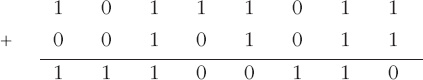Representing and manipulating data in computers

Start this free course now. Just create an account and sign in. Enrol and complete the course for a free statement of participation or digital badge if available.

Free course

# 7.2 Adding 2's complement integers

The leftmost bit at the start of a 2's complement integer (which represents the presence or absence of the weighting −128) is treated in just the same way as all the other bits in the integers. So the rules given at the start of Section 7.1 for adding unsigned integers can be used.

## Example 7

Add the 2's complement integers 1011 1011 and 0010 1011.(Check: 1011 1011 is −69, 0010 1011 is 43 and 1110 0110 is −26; −69 + 43 does equal −26.)

When two negative integers are added, there will be a ninth bit in the result. The extra bit can be ignored; it is a consequence of using bit 7 as a sign bit.

There is, however, a phenomenon that may occur that cannot be ignored. When two negative integers are added the result must be negative and when two positive integers are added the result must be positive. Sometimes the sign bit appears to change as the result of adding two such integers in 2's complement arithmetic. This occurs when the magnitude of the sum is too big for the seven available bits in the data word and so ‘overflows’ into the leftmost bit. The consequence of this is that the addition of two positive integers appears to have produced a negative result, or the addition of two negative integers to have produced a positive result. This phenomenon is called 2's complement overflow.

Consider for example the addition of 0111 1000 (denary 120) and 0000 1111 (denary 15). Performing the addition 0111 1000 + 0000 1111 gives 1000 0111, which looks as if it is a negative number (the leftmost bit is 1). Here is a case where 2's complement overflow has occurred. You can see why this has happened if you note that the result should be 135. This is greater than 127, the maximum positive value that can be represented in an 8-bit word in 2's complement form (see Section 2.4).

Some processors detect that 2's complement overflow has occurred, in which case it is up to the software to deal with it. In other processors the programmer has to build in explicit tests to check that 2's complement overflow has not occurred during additions.